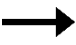# Multiplication Facts StrategiesFact Strategy X 2 Double     2 x 44 + 4 = 8 X 3 Double and add a group 3 x 44 + 4 = 88 + 4 = 12 X 4 Double, double 4 x 44 + 4 = 88 + 8 = 16 X 5 Count by 5s 5 x 45, 10, 15, 20 X 6 Count by 5s and add a group 6 x 45, 10, 15, 2020 + 4 = 24 X 8 Double, double, double 8 x 44 + 4 = 88 + 8 = 1616 + 16 = 32 X 9 Count by 10s and subtract a group 9 x 410, 20, 30, 4040 - 4 = 36